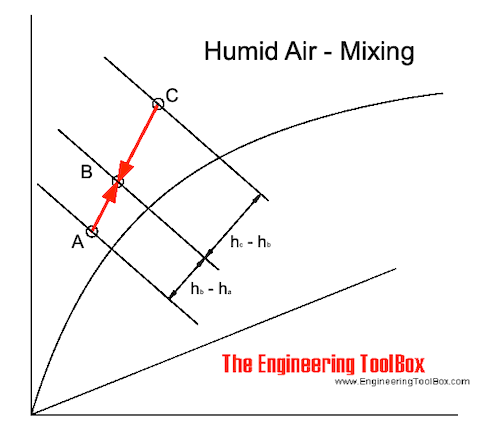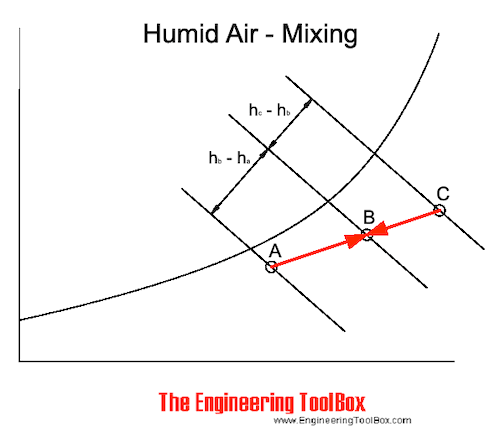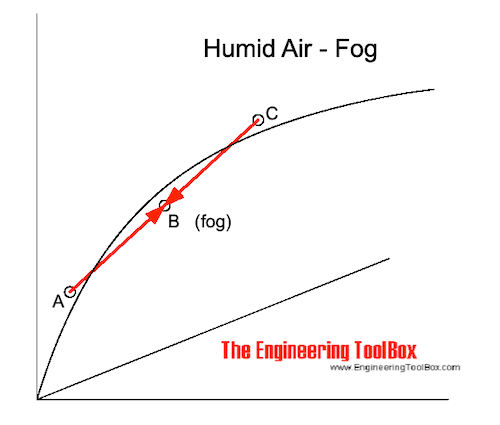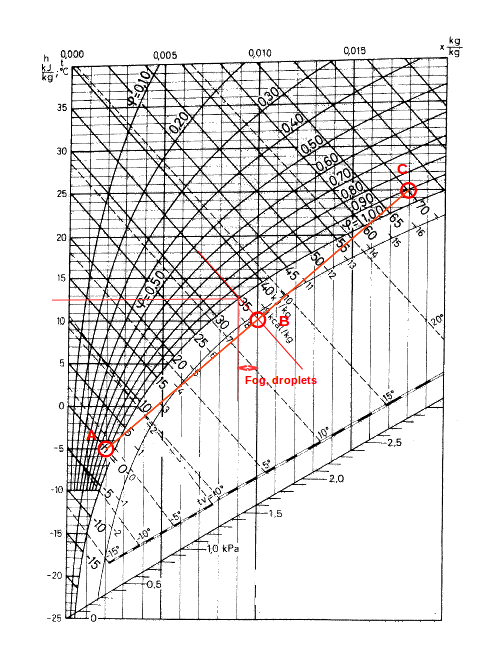Engineering ToolBox - Resources, Tools and Basic Information for Engineering and Design of Technical Applications!

# Mixing of Humid Air

## The change in state wwhen mixing moist air - enthalpy, heat, temperature and specific humidity.

In the Mollier diagram - or in the psychrometric chart - when mixing air of state A and C, the mixing point will be on a straight line in point B.

The position of point B depends on the air volume (or mass) of the air in state A and C.The mixing process are indicated in a Mollier diagram above and in a psychrometric chart below.### Enthalpy Balance when Mixing Air

The heat or enthalphy balance when humid air is mixed can be expressed as:

QA hA + QC hC = (QA + QC) hB                                   (1)

where

Q = volume of the air (m3)

h = enthalpy of the humid air (kJ/m3)

By transforming (1) the enthalpy of the mixed air can be expressed as:

hB = (QA hA + QC hC) / (QA + QC)                                      (1b)

Note! It's common to use air volume when calculating ventilation or air conditioning systems. Using the mass of air is more accurate but within the operation limits of common ventilation systems the volume approach is accurate enough. Be aware that this may not be true in industrial high temperature air handling processes - like dryers or similar.

### Moisture Balance when Mixing Air

The moisture balance when humid air is mixed can be expressed as:

QA xA + QC xC = (QA + QC) xB                                          (2)

where

x = specific humidity or humidity ratio (kgwater/kgdry_air)

By transforming (2) - the specific humidity of the mixed air can be expressed as:

xB = (QA xA + QC xC) / (QA + QC)                                       (2b)

### Humid Air and Fog

When hot humid air is mixed with cold air the result may be fog.

If the mixing point is below the saturation line - as indicated in the figure below - the moisture in the air condensates to small droplets floating in the air.For temperatures below 0oC the water droplets freezes to ice and snow is created.

### Resulting Temperature when Humid Air is Mixed

#### Mixing Point is above the Saturation Line

As long as the mixed air is above the saturation line (no fog in the mix) the temperature balance can be expressed by modifying the enthalpy balance like

QA cpa tA + QC cpa tC = (QA + QC) cpa tB                                           (3)

where

cpa = 1.01 - specific heat capacity of air at constant pressure (kJ/kgoC, kWs/kgK)

The temperature in the mix can be expressed by transforming (3):

tB = (QA tA + QC tC) / (QA + QC)                                               (3b)

#### Mixing Point is below Saturation Line

If the mixing point is below the saturation line - water is condensed as droplets and fog is created.

The amount of condense water can be estimated by following the constant enthalpy line from B to the saturation line. The condensate water is the difference between the specific humidity in point B and in the point where the enthalpy line crosses the saturation line.

The mixed temperature is where the enthalpy line crosses the saturation line.

### Example - Mixing Humid Air

#### Mixing Point is above the Saturation Line

1 m3 of air at 25oC and 50% relative humidity (C) is mixed with 1 m3 air at -5oC and 80% relative humidity (A).

From the Mollier diagram the humidity ratio of (C) is 0.0097 kg/kg. The enthalpy is 50 kJ/kg. The humidity ratio of (A) is 0.002 kg/kg. The enthalpy is 0 kJ/kg.

The humidity ratio of the mixture can be calculated like

xB = (QA xA + QC xC) / (QA + QC)                                             (4)

= ((1 kg) (0.002 kg/kg) + (1 kg) (0.0097 kg/kg) ) / ((1 kg) + (1 kg))

= 0.0058 kg/kg

The enthalpy of the mixed air can be calculated like

hB = ((1 kg) (0 kJ/kg) + (1 kg) (50 kJ/kg)) / ((1 kg) + (1 kg))

= 25 kJ/kg

The temperature of the mixed air can be calculated as:

tB = ((1 kg) (-5oC) + (1 kg) (25oC)) / ((1 kg) + (1 kg))

= 10oC

Based on the information above and the Mollier diagram - the relative humidity in the mixing point can be estimated to approximately 80%.

#### The Mixing Point is below the Saturation Line

1 m3 of air at 25oC and 90% relative humidity (C) is mixed with 1 m3 air at -5oC and 80% relative humidity (A).

From the Mollier diagram the humidity ratio of (C) is 0.018 kg/kg. The enthalpy is 70 kJ/kg. The humidity ratio of (A) is 0.002 kg/kg. The enthalpy is 0 kJ/kg.

The enthalpy of the mixture can be calculated as:

hB = ((1 kg) (0 kJ/kg) + (1 kg) (70 kJ/kg)) / ((1 kg) + (1 kg))

= 35 kJ/kg

The humidity ratio of the mixture can be calculated as:

xB = ((1 kg) (0.002 kg/kg) + (1 kg) (0.018 kg/kg)) / ((1 kg) + (1 kg))

= 0.01 kg/kg

Note! This is the humidity ratio including the water droplets - the fog.

The "virtual" mixing point in the Mollier diagram is where the enthalpy line - 35 kJ/kg and the humidity ratio line - 0.01 kg/kg - intersect.

The temperature in the mixed air can be found in the diagram where the enthalpy line - 35 kJ/kg - crosses the saturation line. From the Mollier diagram the mixed temperature is approximately 12.7 oC.

According the Mollier diagram the moisture ratio at this point is approximately  0.0089 kg/kg. The moisture created as fog or droplets is

(0.01 kg/kg) - (0.0089 kg/kg)

= 0.0011 kg/kg## Related Topics

• Air Psychrometrics - Moist and humid air - psychrometric charts, Mollier diagrams, air-condition temperatures and absolute and relative humidity and moisture content.

## Engineering ToolBox - SketchUp Extension - Online 3D modeling!

Add standard and customized parametric components - like flange beams, lumbers, piping, stairs and more - to your Sketchup model with the Engineering ToolBox - SketchUp Extension - enabled for use with the amazing, fun and free SketchUp Make and SketchUp Pro .Add the Engineering ToolBox extension to your SketchUp from the SketchUp Pro Sketchup Extension Warehouse!

Translate

## Privacy

We don't collect information from our users. Only emails and answers are saved in our archive. Cookies are only used in the browser to improve user experience.

Some of our calculators and applications let you save application data to your local computer. These applications will - due to browser restrictions - send data between your browser and our server. We don't save this data.

## Citation

• Engineering ToolBox, (2004). Mixing of Humid Air. [online] Available at: https://www.engineeringtoolbox.com/mixing-humid-air-d_694.html [Accessed Day Mo. Year].

Modify access date.

. .

#### Scientific Online Calculator3 30